#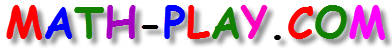The 4th grade math games on this website focus on several important topics such as adding and subtracting decimals and fractions with common denominators, rounding decimals, measuring angles with appropriate units and tools, understanding the characteristics of geometric plane and solid figures, using common measurement units to determine weight, and using tables, graphs, and charts to record and analyze data.

Fourth grade students, are you ready to show off your math skills? Try one of these games!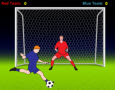Properties of Addition - Soccer Game

In this fun soccer math game, 4th grade students will identify and use different properties of addition.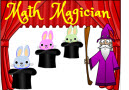Math Magician Multiples Game

Match the multiples on the bunnies with the correct solutions on the hats in this fun Math Magician Multiples Game.Division Halloween Math Game (New)

Blast off into math fun by destroying halloween monsters when solving these division problems. You will divide whole numbers with and sometimes without remainders.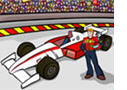Order and Compare Numbers to 100

Math racing game about ordering and comparing two-digit whole numbers.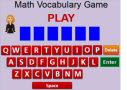4th Grade Vocabulary Game

This is a fun math vocabulary game that 4th grade students can use to review important math terms and definitions.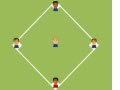Time Change Baseball Game
Practice telling time when adding hours to times shown in this exciting Baseball Math Time Change Game.Math Magician Factors Game

Match the factors on the bunnies with the correct solutions on the hats in this fun Math Magician Factors Game.Dividing by One-Digit Numbers

This is an exciting multi-player baseball game. Solve division problems correctly for a chance to score homeruns.Math Racing - Place Value Game

In this fast-paced math racing game, 4th grade students will identify large whole numbers in standard and word form.Measurement Baseball Game
Play this fun and exciting Baseball Math Measurement Game to help with your learning about customary and metric measurements.4th Grade Halloween Math Game (New)

Practice your math skills and have fun destroying different monsters when solving problems with different numbers written in standard or expanded form.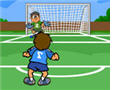Adding Decimals Soccer Game

In this fun game, kids will practice adding decimals and earn tons of points by scoring goals.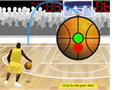Adding Decimals Basketball GameAdding Money Game

Your kids can practice solving word problems about adding money by playing this interesting game. The game has a virtual cash register.Baseball Math - Adding Decimals Game

Elementary students can practice adding decimals by playing this interactive baseball math game.Soccer Math Game - Subtracting Decimals

Kids must solve each subtraction problem correctly to have a chance to kick the ball and score points.Baseball Math - Subtracting Decimals

Students must hit a homerun to get to answer a problem about subtracting decimals. When playing this game, kids will earn points for hitting a homerun and for correctly answering different math problems.Basketball Math - Subtracting Decimals

This is an exciting basketball game for young students. They can play it alone, with another friend, or even in teams.Subtracting Money Game

4th grade students can practice solving word problems about subtracting money by playing this interactive game.Basketball Multiplication Game

In this fun basketball game, fourth grade students will multiply one-digit factors with two-digit whole numbers.Division With Remainder Game (New)

In this fun online soccer game, 4th grade students will solve division with remainder problems.

Return from the 4th Grade Math Games page to the Elementary Math Games page or to the Math Play homepage.